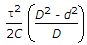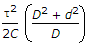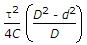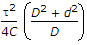# Mechanical Engineering - Strength of Materials

### Exercise :: Strength of Materials - Section 7

21.

The strain energy stored in a hollow circular shaft of outer diameter (D) and inner diameter (d) subjected to shear stress is

 A.x Volume of shaft B.x Volume of shaft C.x Volume of shaft D.x Volume of shaft

Explanation:

No answer description available for this question. Let us discuss.

22.

Fatigue test is carried out for

 A. stresses varying between two limits of equal value, but of opposite sign B. stresses varying between two limits of unequal value, but of opposite sign C. stresses varying between two limits of unequal value but of same sign D. all of the above

Explanation:

No answer description available for this question. Let us discuss.

23.

The section modulus (Z) of a beam is given by

 A. I/y B. I.y C. y/I D. M/I

Explanation:

No answer description available for this question. Let us discuss.

24.

Every direct stress is always accompanied by a strain in its own direction and an opposite kind of strain in every direction, at right angles to it. Such a strain is known as

 A. linear strain B. lateral strain C. volumetric strain D. shear strain

Explanation:

No answer description available for this question. Let us discuss.

25.

The shear force of a cantilever beam of length l and carrying a gradually varying load from zero at the free end and w per unit length at the fixed end is __________ at the fixed end.

 A. zero B. wl/4 C. wl/2 D. wl